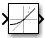# Rate Limiter

Limit rate of change of signal

•Libraries:

## Description

The Rate Limiter block limits the first derivative of the signal passing through it. The output changes no faster than the specified limit. The derivative is calculated using this equation:

`$rate=\frac{u\left(i\right)-y\left(i-1\right)}{t\left(i\right)-t\left(i-1\right)}$`

where u(i) and t(i) are the current block input and time, and y(i-1) and t(i-1)) are the output and time at the previous step. The output is determined by comparing rate to the Rising slew rate and Falling slew rate parameters:

• If rate is greater than the Rising slew rate parameter (R), the output is calculated as

`$y\left(i\right)=\Delta t\cdot R+y\left(i-1\right).$`

• If rate is less than the Falling slew rate parameter (F), the output is calculated as

`$y\left(i\right)=\Delta t\cdot F+y\left(i-1\right).$`

• If rate is between the bounds of R and F, the change in output is equal to the change in input:

`$y\left(i\right)=u\left(i\right)$`

When the block is running in continuous mode (for example, Sample time mode is `inherited` and Sample time of the driving block is zero), the Initial condition is ignored. The block output at `t = 0` is equal to the initial input:

`$y\left(0\right)=u\left(0\right)$`

When the block is running in discrete mode (for example, Sample time mode is `inherited` and Sample time of the driving block is nonzero), the Initial condition is preserved:

`$y\left(-1\right)=Ic$`

where Ic is the initial condition. The block output at ```t = 0``` is calculated as if rate is outside the bounds of R and F. For `t = 0`, rate is calculated as follows:

`$rate=\frac{u\left(0\right)-y\left(-1\right)}{sample\text{\hspace{0.17em}}time}$`

## Ports

### Input

expand all

The input signal to the rate limiter algorithm.

Data Types: `single` | `double` | `int8` | `int16` | `int32` | `int64` | `uint8` | `uint16` | `uint32` | `uint64` | `fixed point`

### Output

expand all

Output signal from the rate limiter algorithm.

Data Types: `single` | `double` | `int8` | `int16` | `int32` | `int64` | `uint8` | `uint16` | `uint32` | `uint64` | `fixed point`

## Parameters

expand all

Specify the limit of the rising rate of the input signal. This parameter is tunable for fixed-point inputs.

#### Programmatic Use

 Block Parameter: `RisingSlewLimit` Type: character vector Values: real number Default: `'1'`

Specify the lower limit on the falling rate of the input signal. This parameter is tunable for fixed-point inputs.

#### Programmatic Use

 Block Parameter: `FallingSlewLimit` Type: character vector Values: real number Default: `'-1'`

Specify the sample time mode, `continuous` or `inherited` from the driving block.

#### Programmatic Use

 Block Parameter: `SampleTimeMode` Type: character vector Values: `'inherited'` | `'continuous'` | Default: `'inherited'`

Set the initial output of the simulation. Simulink® does not allow you to set the initial condition of this block to `inf` or `NaN`.

#### Programmatic Use

 Block Parameter: `InitialCondition` Type: character vector Values: `scalar` Default: `'0'`

Select this check box to cause the commands to treat the gain as 1. The linearization commands in Simulink software treat this block as a gain in state space. Clear the box to have the commands treat the gain as 0.

#### Programmatic Use

 Block Parameter: `LinearizeAsGain` Type: character vector Values: `'off'` | `'on'` Default: `'on'`

## Block Characteristics

 Data Types `double` | `fixed point` | `integer` | `single` Direct Feedthrough `yes` Multidimensional Signals `no` Variable-Size Signals `no` Zero-Crossing Detection `no`

## Version History

Introduced before R2006a